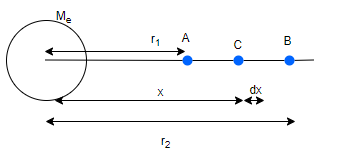# Gravitational Potential energy

## Gravitational Potential Energy

• Potential energy of a body is the energy stored in it at its given position
• Change in Potential energy occurs when force act on the body and this change in PE is equal to the workdone on the body by force applied
• if workdone by a force does not depend on path taken , it is called conservative forces
• Gravitational force or Force of gravity is an example of conservative forces
• Potential energy due to gravitational force is called gravitational potential energy
• Consider the earth particle system in which particle of mass is raised at height h above the earth surface . Change in Gravitational Potential energy is $U_F-U_i = mgh$
Where force f=mg is treated as constant

## Derivation of Gravitational Potential Energy

• Lets check out the general expression for change in gravitational potential energy
•• Consider a body of mass m situated at point A at a distance $r_1$ from earth's center and it is moved from point A to B at distance $r_2$
• if at any instant body is at point C where OC=x,the gravitational force on body at C is
$F= \frac {GM_e m}{x^2}$
• Therefore workdone in moving body from C to B is
$dW=-Fdx$
$dW= -\frac {GM_e m}{x^2} dx$
• Now change in Potential energy is given by
$dU=-dW$
The increase in Potential energy in bringing body from $r_1$ to $r_2$ is
$\Delta U= \int_{r_1 }^{r_2} \frac {GM_em}{x^2} dx$
$\Delta U= GM_e m(\frac {1}{r_1} - \frac {1}{r_2})$
or
$U(r_2) - U(r_1) = GM_e m (\frac {1}{r_1} - \frac {1}{r_2})$
• if we choose Potential energy at infinity be zero,then
Then moving from distance r to infinity
$U(\infty) - U(r) = \frac {GM_em}{r}$
Now since $U(\infty)=0$
$U(r) = - \frac {GM_e m}{r}$
• Therefore the Gravitational Potential energy of the earth mass system is given by
$U=- \frac {GM_e m}{r}$
Where r is the distance between earth and the body
• We have proof for earth mass system but this is true for any two mass system
Therefore , In general Gravitational Potential energy between two particle system is
$U=- \frac {Gm_1 m_2}{r}$
Where $m_1$ and $m_2$ are the masses and r is the seperation between them . Potential is assumed to be zero at infinite speration

## Gravitational Potential Energy of system of Particles

Total potential energy will be equal the the sum of energies (given by the above equation) for all possible pairs of its constituent particles. This is an example of the application of the superposition principle.
$U=U_1 + U_2 + U_3---$